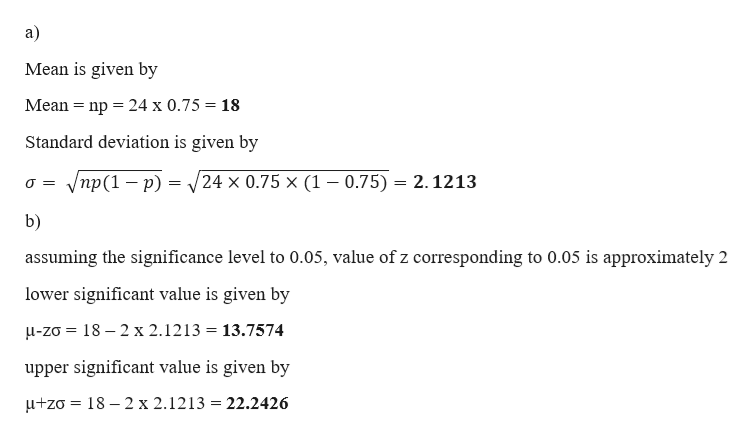# Assume that hybridization experiments are conducted with peas having the property that for​ offspring, there is a0.75probability that a pea has green pods. Assume that the offspring peas are randomly selected in groups of24.Complete parts​ (a) through​ (c) below.a. Find the mean and the standard deviation for the numbers of peas with green pods in the groups of24. The value of the mean ismuμequals=peas.​(Type an integer or a decimal. Do not​ round.)The value of the standard deviation issigmaσequals=peas.​(Round to one decimal place as​ needed.)b. Use the range rule of thumb to find the values separating results that are significantly low or significantly high. Values ofpeas or fewer are significantly low.​(Round to one decimal place as​ needed.)Values ofnothingpeas or greater are significantly high.​(Round to one decimal place as​ needed.)c. Is a result of11peas with green pods a result that is significantly​ low? Why or why​ not? The result▼ is notissignificantly​ low, because11peas with green pods is▼ less thangreater thanequal tonothingpeas.

Question
145 views
Assume that hybridization experiments are conducted with peas having the property that for​ offspring, there is a
0.75
probability that a pea has green pods. Assume that the offspring peas are randomly selected in groups of
24.
Complete parts​ (a) through​ (c) below.
a. Find the mean and the standard deviation for the numbers of peas with green pods in the groups of
24.

The value of the mean is
muμequals=
peas.
​(Type an integer or a decimal. Do not​ round.)
The value of the standard deviation is
sigmaσequals=
peas.
​(Round to one decimal place as​ needed.)
b. Use the range rule of thumb to find the values separating results that are significantly low or significantly high.

Values of
peas or fewer are significantly low.
​(Round to one decimal place as​ needed.)
Values of
nothing
peas or greater are significantly high.
​(Round to one decimal place as​ needed.)
c. Is a result of
11
peas with green pods a result that is significantly​ low? Why or why​ not?

The result

is not
is
significantly​ low, because
11
peas with green pods is

less than
greater than
equal to
nothing
peas.
check_circle

Step 1

Given data

probability that a pea has green pods is (p) = 0.75

group size (n) = 24help_outlineImage Transcriptionclosea) Mean is given by Mean nр %3D24 х 0.75 3 18 Standard deviation is given by /пр (1 — р) - 24 x 0.75 x (1 - 0.75) 2.1213 O= b) assuming the significance level to 0.05, value of z corresponding to 0.05 is approximately 2 lower significant value is given by u-zo 18 2 x 2.1213 = 13.7574 upper significant value is given by 18-2 x 2.1213 = 22.2426 +zo fullscreen
Step 2

c)

From the value of lower significant value

11 peas with green pods is significantly lowe...

### Want to see the full answer?

See Solution

#### Want to see this answer and more?

Solutions are written by subject experts who are available 24/7. Questions are typically answered within 1 hour.*

See Solution
*Response times may vary by subject and question.
Tagged in

### Other